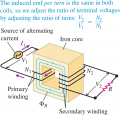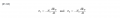# Working principles of AC transformers

#### x11

Joined Dec 25, 2019
6
I am currently learning about transformers in my physics class.How does mutual inductance even apply here? The coils act as solenoids, and the field outside of a solenoid is 0, meaning that no emf should be induced in the opposing coil. We're also given equations such as,The textbook claims that "The flux per turn ΦB is the same in both the primary and the secondary", which also doesn't make sense to me. How can the flux per turn be the same when the voltage is different in both circuits?

#### ronsimpson

Joined Oct 7, 2019
2,747
The textbook claims that "The flux per turn ΦB is the same in both the primary and the secondary", which also doesn't make sense to me. How can the flux per turn be the same when the voltage is different in both circuits?
The voltage/turn is the same on both sides. (not the volts but the V/T)

#### nsaspook

Joined Aug 27, 2009
11,743

#### crutschow

Joined Mar 14, 2008
32,846
Also note that the flux is proportional to the primary volts (and inversely with frequency), not the current.
The flux value is basically independent of load current.

Last edited: